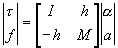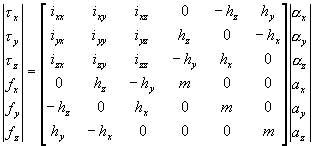# Physics - Forces and Inertia

The previous page discussed momentum and how it is conserved in a closed system. This page is about how momentum can be exchanged between bodies by means of forces.

Not all forces transfer momentum, in some cases the forces may be in balance and therefore do not effect the dynamics. (see statics pages). If the forces are not in balance, then momentum will be transferred, this will create a force (inertia) which is equal and opposite to the sum of forces on the object.

In a collision between two objects the momentum can be exchanged very quickly (very large force in a very short time) in this case we call it an impulse as described here.

## Newtons Second Law

A property of mass is its resistance to a change of velocity. This is quantified by Newtons second law which says that the acceleration is:

• Proportional to the net external force.
• Inversely proportional to its mass.
• In the direction of the force.

In other words:

F=m a

 where: other definitions symbol description type units F net force vector kg m/s2 m mass scalar kg a acceleration vector m/s2

There is also an equivalent for rotation:

 τa = [Ia]α or in inertial fixed frame (varies with time) τr = [Ir]α + ω×[Ir]ω in body coordinates (inertia tensor fixed)
 where: other definitions symbol description type units τa torque in absolute coordinates (body being moved relative to ground) bivector N m τr torque in relative coordinates (control surfaces or jets on body) bivector N m [Ia] inertia tensor in absolute coordinates (this will vary with orientation and since the body is rotating the elements of the tensor will vary with time) tensor kg m2 [Ir] inertia tensor in relative coordinates (since this is relative to the body it will be constant) tensor kg m2 α angular acceleration bivector s-2

The first form of the equation looks simplest but the values vary with time and therefore the second form can be easier to use. Since it is, generally, very difficult to continuously recalculate the changing inertia matrix as seen from an inertial fixed frame, the conservation of angular momentum is most frequently written in a coordinate frame fixed in the rotating rigid body.

If the rotation vector is aligned with a principal axis of the rotating body then the term: ω×[Ir]ω becomes zero, the term: ω×[Ir]ω describes the behavior known as gyroscopic or processional motion.

 Tensor- A set of components that obeys some transformation law in n-dimentional space. more

When working in 3 dimensions, then F, a, T andcan be represented as vectors and m and I as matrices.

For example, then the equations become:

F=m a
 Fx Fy Fz
=
 m 0 0 0 m 0 0 0 m
 ax ay az
τ = [I] α
 τx τy τz
=
 ixx ixy ixz iyx iyy iyz izx izy izz
 αx αy αz

where x,y,z are the mutually perpendicular coordinate directions. Note that mass, m is the same in all directions whereas the coordinates of the inertia matrix depend on the orientation of the object and the individual elements represent the volume integrals as follows:

ixx = ∫ ∫ ∫ (y2 + z2) p dv
ixy = - ∫ ∫ ∫ (x y) p dv
ixz = - ∫ ∫ ∫ (x z) p dv
iyx = - ∫ ∫ ∫ (y x) p dv
iyy = ∫ ∫ ∫ (x2 + z2) p dv
iyz = - ∫ ∫ ∫ (y z) p dv
izx = - ∫ ∫ ∫ (z x) p dv
izy = - ∫ ∫ ∫ (z y) p dv
izz = ∫ ∫ ∫ (x2 + y 2) p dv

## 6d vectors

So far the linear and angular components have been considered separately, however they are related, so it is useful to combine them. The method shown here uses the Featherstone's spatial vector notation (see http://www.syseng.anu.edu.au/~roy/technical.html) Thank you to Michael Shantz telling me about this (see hybridgdc.pdf file at http://michaelshantz.com ).

In this notation, the above equations are combined together as follows:So when working in 3 dimensional space this can be expanded out to become the Newton-Euler equations of motion (since Newton originated the linear and Euler the angular).where: other definitions symbol description type units h m * s = mass times distance vector Kg * m τ torque bivector Kg m2/s2 f force vector Kg m/s2 α angular acceleration bivector m-2 a linear acceleration vector m/s2 i inertia Kg m2

The explanation for these h terms is because, when a solid object is rotating and contacts another object at a point offset from its axis of rotation, then linier forces can be converted into torques and visa versa.When a force is appled to a free floating rigid object, then an equal and opposite force is produced due to inertia. Since the two forces are offset by distance 's' then this will produce a turning moment.

This torque is equal to the force times its perpendicular distance from the centre of rotation.

τ = r × F

 where: other definitions symbol description type units τ torque bivector N m r distance from the centre of rotation vector m F the force vector N × the cross product vector operator

substituting F=m a (assuming m is constant) gives,

τ = r × m a

therefore defining h = m *gives

τ= h ×a

In the 3d case T, h and a are vectors and × is the cross product:

 τx τy τz
=
 0 -hz hy hz 0 -hx -hy hx 0
 ax ay az

This give the h terms in the full matrix

 metadata block see also: Correspondence about this page Book Shop - Further reading. Where I can, I have put links to Amazon for books that are relevant to the subject, click on the appropriate country flag to get more details of the book or to buy it from them.Game Physics - This book has some useful stuff, its more of a textbook, not a step by step guide (although it does have a disc with a lot of C++ code). About the first third of the book is a physics textbook with theoretical exercises, the middle bit covers physics engine topics, and the last third of the book covers mathematical topics. I think I would use this book as a reference book to lookup the theory behind something I might be working on rather than a book to work through in order.Robot Dynamics Algorithms (Kluwer International Series in Engineering and Computer Science, 22) Commercial Software Shop Where I can, I have put links to Amazon for commercial software, not directly related to the software project, but related to the subject being discussed, click on the appropriate country flag to get more details of the software or to buy it from them.Mathmatica

This site may have errors. Don't use for critical systems.